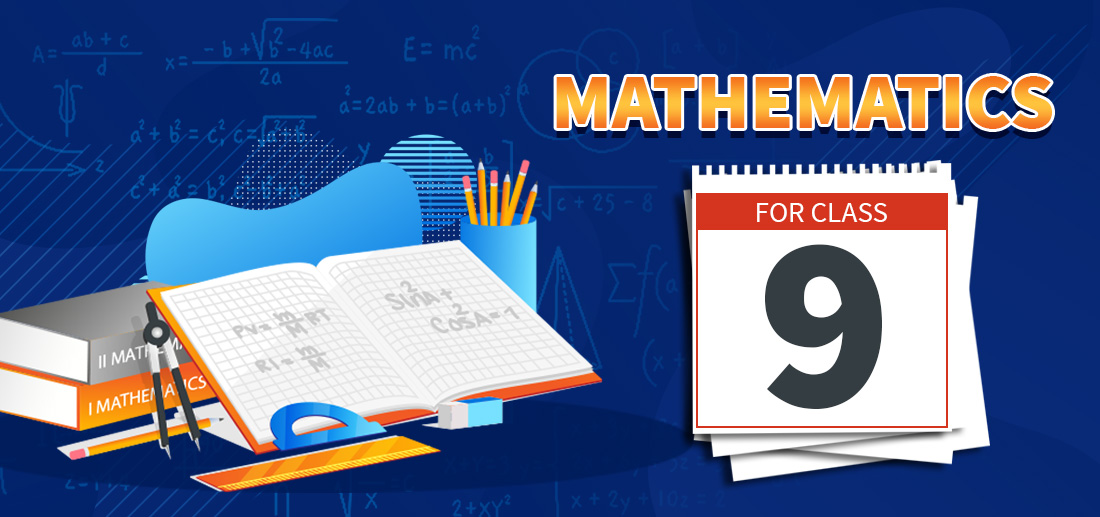# CBSE Class 9 Maths Notes

• Last Updated : 27 May, 2021

CBSE Class 9 Mathematics Concepts, makes students to understand all the concepts of class 9 maths chapter-wise as it covers all the topics and subtopics provided in the syllabus of class 9 maths. GeeksforGeeks provided the following chapter-wise notes that contain numbers of theorems with their proper explanations, formulae with their applications, and graphs that need to accelerate students’ knack of practicing problems and boost their interest to pursue maths in higher classes.Attention reader! All those who say programming isn't for kids, just haven't met the right mentors yet. Join the  Demo Class for First Step to Coding Coursespecifically designed for students of class 8 to 12.

The students will get to learn more about the world of programming in these free classes which will definitely help them in making a wise career choice in the future.

### Chapter 1: Number Systems

The numeral or number system is the combination of natural, integers, rational, irrational, and real numbers. This lesson covers the entire concepts of the numeral system and its types, representation on the number line, laws of rational exponents, and integral powers.

### Chapter 2: Polynomials

Polynomial is an expression that comprises variables and coefficients which involves the operations like addition, subtraction, multiplication, and non-negative integer exponentiation of variables.

### Chapter 3: Coordinate Geometry

The coordinate geometry is a part of geometry where the position of the points on the plane is described with the help of an ordered pair of numbers called coordinates.

### Chapter 4: Linear Equations in Two Variables

Any equation which can be defined in the form ax + by + c = 0, where a, b and c are real numbers, and a and b are not both zero, is called a linear equation in two variables.

### Chapter 5: Introduction to Euclid’s Geometry

Euclidean geometry is the branch of geometry that deals with the study of geometrical shapes and figures based on different axioms and theorems. This study basically provides a brief explanation for flat surfaces.

### Chapter 6: Lines and Angles

In geometry, Lines and Angles are defined as figures that are made up of infinite points extending indefinitely in both directions. Lines are straight and have length and breadth, while an angle is a figure from which two rays emerge from a common point.

### Chapter 7: Triangles

Geometrically, a triangle is defined as a three-sided polygon consist of three edges and three vertices. The most important and applied property of a triangle is its Angle sum property which means the sum of the internal angles of a triangle is equal to 180 degrees only.

A quadrilateral is a plane geometrical figure which has four sides and has four corners or vertices. Typically, quadrilaterals are rectangle, square, trapezoid, and kite or irregular and uncharacterized figures with four sides.

### Chapter 9: Areas of Parallelograms and Triangles

The area of a plane figure is described as the amount of the planar surface covered by a closed geometric figure like rectangle, square, etc. The area of a parallelogram is equal to the product of its

### Chapter 10: Circles

A circle is a geometrical shape which is defined as the locus of points that moves in a plane so that its distance from a fixed point is always constant. This fixed point is the Centre of the circle while the fixed distance from it is called the radius of the circle.

### Chapter 11: Constructions

Construction helps to understand the approach to construct different types of triangles for different given conditions using a ruler and compass of required measurements.

### Chapter 12: Heron’s Formula

In this chapter, a formula called Heron’s formula is introduced which helps to determine the area of the triangle when three sides of its being given. The application of this formula also helps to find the area of other different polygons also.

### Chapter 13: Surface Areas and Volumes

Surface area and volume are the measures calculated for a three-dimensional geometrical shape like a cube, cuboid, sphere etc. The surface area of any given object is the area occupied by the surface of the object while volume is the amount of space available in an object.

### Chapter 14: Statistics

Statistics is the study of the representation, collection, interpretation, analysis, presentation, and organization of data. In other words, it is a mathematical way to collect, summarize data. The representation of data differently along with the frequency distribution.

### Chapter 15: Probability

The Probability in this class includes basic probability theory, which is also used in the probability distribution, to learn the possibility of outcomes for a random experiment and to find the probability of a single event to occur, when the total number of possible outcomes.

My Personal Notes arrow_drop_up